# Bayesian Modeling with RJAGS¶

## Libraries¶

In :
options(warn = -1)
library(ggplot2)
library(ggridges)
library(ggExtra)
library(dplyr)
library(rjags)
library(coda)
library(openintro)
library(mosaic)


## Simulating a Beta prior¶

Suppose you're running in an election for public office. Let $p$ be your underlying support, the proportion of voters that plan to vote for you. Based on past polls, your prior model of $p$ is captured by a Beta distribution with shape parameters $45$ and $55$.

We will approximate the $p \sim Beta(45, 55)$ prior using random samples from the rbeta() function.

In :
# Sample 10000 draws from Beta(45,55) prior
prior_A <- rbeta(n = 10000, shape1 = 45, shape2 = 55)

# Store the results in a data frame
prior_sim <- data.frame(prior_A)

# Construct a density plot of the prior sample
ggplot(prior_sim, aes(x = prior_A)) + geom_density(color='darkblue', fill = 'lightblue') + theme_ridges()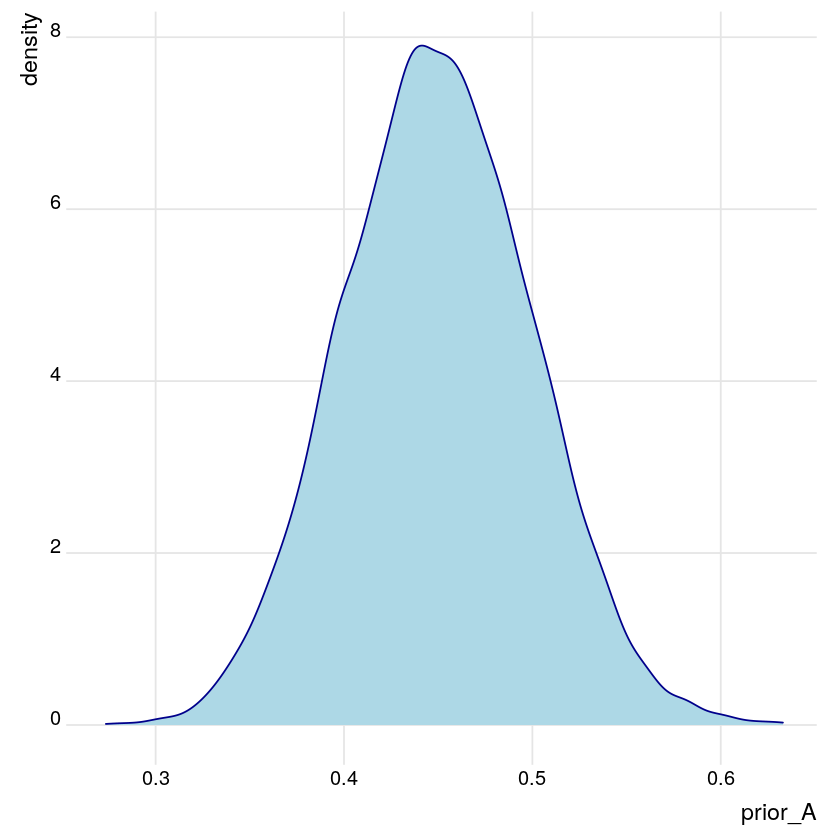## Comparing & contrasting Beta priors¶

The $Beta(a,b)$ distribution is defined on the interval from $0$ to $1$, thus provides a natural and flexible prior for your underlying election support, $p$. We can tune the Beta shape parameters $a$ and $b$ to produce alternative prior models. We will compare our original $Beta(45,55)$ prior with two alternatives: $Beta(1, 1)$ and $Beta(100, 100)$.

In :
# Sample 10000 draws from the Beta(1,1) prior
prior_B <- rbeta(n = 10000, shape1 = 1, shape2 = 1)

# Sample 10000 draws from the Beta(100,100) prior
prior_C <- rbeta(n = 10000, shape1 = 100, shape2 = 100)

# Combine the results in a single data frame
prior_sim <- data.frame(samples = c(prior_A, prior_B, prior_C),
priors = rep(c("A","B","C"), each = 10000))

# Plot the 3 priors
ggplot(prior_sim, aes(x = samples, fill = priors)) + geom_density(alpha = 0.5) + theme_ridges()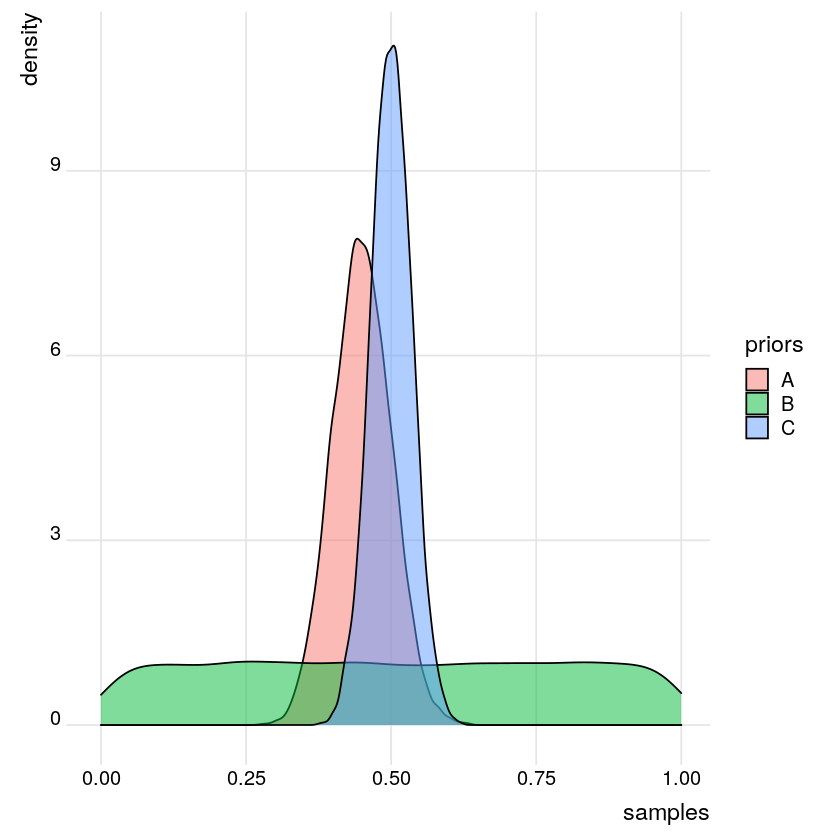Prior $B$ reflects vague prior information about $p$ - it gives equal prior weight to all values of $p$ between $0$ and $1$.

Prior $C$ reflects more prior certainty about $p$ - it has less spread and is centered around a mean that's greater than that for Prior $A$.

## Polling Data¶

• parameter
• $p$ = proportion of voters that support you
• data
• $X = 6$ of $n = 10$ or $60\%$ of polled voters plan to vote/support you
• Insights
• You are more likely to have observed these data if $p \approx 0.6$ than if $p < 0.5$.

## Modeling the dependence of $X$ on $p$¶

• Poll assumptions:

• Voters are independent: $p$ = probability that a voter supports you

• $X$ = number of $n$ polled voters that support you (count of successes in $n$ independent trials, each having probability of success $p$)
• Conditional distribution of $X$ given $p$:
$$X \sim Bin(n, p)$$

## Likelihood¶

The likelihood function summarizes the likelihood of observing polling data $X$ under different values of the underlying support parameter $p$. It is a function of $p$. Thus the likelihood is a function of $p$ that depends on the observed data $X$, in turn it provides insight into which parameter values are most compatible with the poll.

• high likelihood $\Rightarrow p$ is compatible with the data

• low likelihood $\Rightarrow p$ is not compatible with the data

# Simulating the dependence of $X$ on $p$¶

In your quest for election to public office, your campaign polls $10$ likely voters. Let $X$ be the number that support you. Of course, $X$ varies from sample to sample and depends upon $p$, your underlying support in the broader population. Since $X$ is a count of successes in $10$ independent trials, each having probability of success $p$, we can model its dependence on $p$ by the Binomial distribution: $Bin(10, p)$.

We will simulate the Binomial model using random samples from the rbinom(n, size, prob) function. This vectorized function draws $n$ samples from a $Bin(size, prob)$ distribution. Given a vector of prob values, the first prob value will be used for the first draw, the second prob value will be used for the second draw, etc.

In :
# Define a vector of 1000 p values from 0 to 1
p_grid <- seq(from = 0, to = 1, length.out = 1000)

# Simulate 1 poll result for each p in p_grid
poll_result <- rbinom(n = 1000, # Number of simulations
size = 10, # Number of Trials (voters)
prob = p_grid) # Underlying proportion of success between 0.0 and 1.0

# Create likelihood_sim data frame
likelihood_sim <- data.frame(p_grid, poll_result)

# Density plots of p_grid grouped by poll_result
ggplot(likelihood_sim, aes(x = p_grid, y = poll_result, group = poll_result, fill = poll_result, alpha = 0.8)) +
geom_density_ridges() + theme_ridges()

Picking joint bandwidth of 0.0412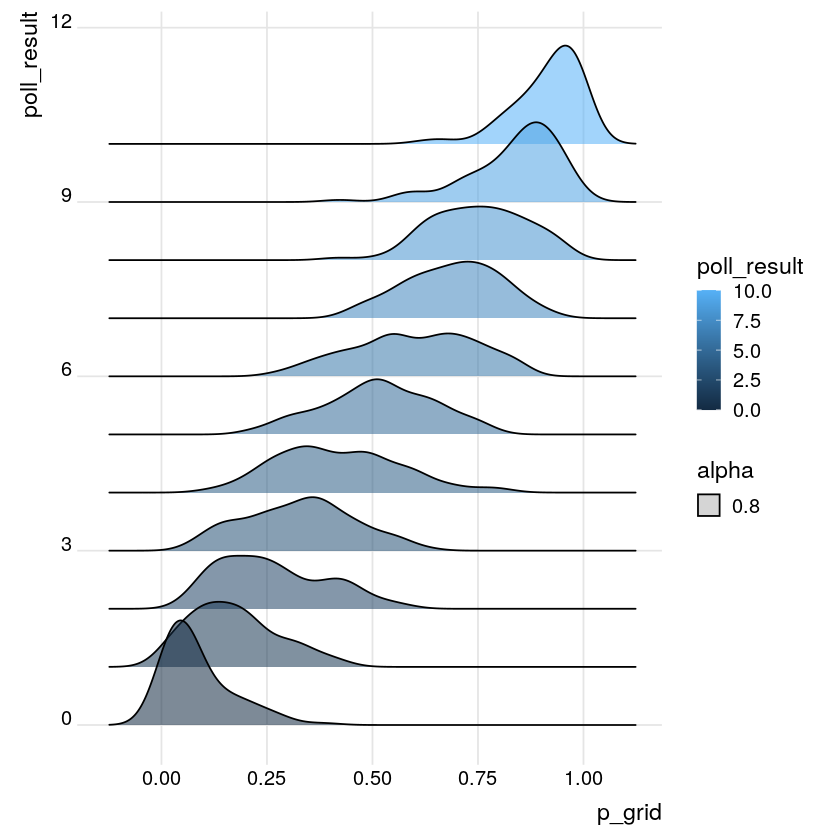Notice that polls in which $0$ people supported you (poll_result = 0) correspond to smaller values of underlying support $p$ (p_grid). The opposite is true for polls in which all $10$ people supported you.

## Approximating the likelihood function¶

The first election poll is in! $X = 6$ of $10$ polled voters plan to vote for you. You can use these data to build insight into your underlying support $p$. To this end, we will use the likelihood_sim data frame. This contains the values of $X$ (poll_result) simulated from each of $1,000$ possible values of $p$ between $0$ to $1$ (p_grid).

Here we supply a fill condition in order to highlight the distribution which corresponds to our observed poll_result, $X=6$. This provides insight into which values of $p$ are the most compatible with our observed poll data.

In :
# Density plots of p_grid grouped by poll_result
ggplot(likelihood_sim, aes(x = p_grid, y = poll_result, group = poll_result, fill = poll_result == 6, alpha = 0.8)) +
geom_density_ridges() + theme_ridges()

Picking joint bandwidth of 0.0412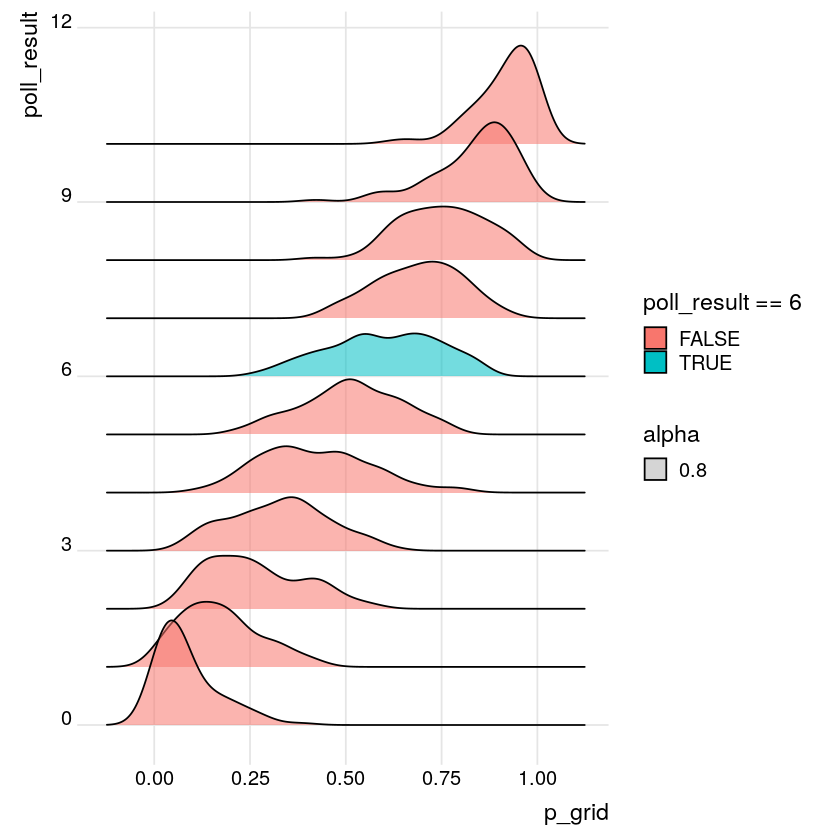This is a scaled approximation of the likelihood function. It indicates that the simulated surveys in which $6$ of $10$ voters supported you corresponded to underlying support $p$ that ranged from approximately $0.25$ to $1$, with $p$ around $0.6$ being the most common.

# Posterior model of $p$¶

• Prior: $p \sim Beta(45, 55)$

• likelihood: $X \sim Bin(10, p)$

• Bayes' Rule: posterior $\propto$ prior $\times$ likelihood

# Getting Started with RJAGS¶

Bayesian Models in RJAGS: DEFINE

• $X \sim Bin(n, p)$
• $p \sim Beta(a, b)$

## Define, compile, and simulate¶

In your election quest, let $p$ be the proportion of the underlying voting population that supports you. Built from previous polls & election data, your prior model of $p$ is a $Beta(a,b)$ with shape parameters $a=45$ and $b=55$. For added insight into $p$, you also polled $n$ potential voters. The dependence of $X$, the number of these voters that support you, on $p$ is modeled by the $Bin(n,p)$ distribution.

In the completed poll, $X=6$ of $n=10$ voters supported you. The next goal is to update the model of $p$ in light of these observed polling data. To this end, we will use the rjags package to approximate the posterior model of $p$. We break this down into the $3$ rjags steps: define, compile, simulate.

In :
# DEFINE the model
vote_model <- "model{
# Likelihood model for X
X ~ dbin(p, n)

# Prior model for p
p ~ dbeta(a, b)
}"

# COMPILE the model
vote_jags <- jags.model(textConnection(vote_model),
data = list(a = 45, b = 55, X = 6, n = 10),
inits = list(.RNG.name = "base::Wichmann-Hill", .RNG.seed = 100))

# SIMULATE the posterior
vote_sim <- coda.samples(model = vote_jags, variable.names = c("p"), n.iter = 10000)

# PLOT the posterior
plot(vote_sim, trace = FALSE)

Compiling model graph
Resolving undeclared variables
Allocating nodes
Graph information:
Observed stochastic nodes: 1
Unobserved stochastic nodes: 1
Total graph size: 5

Initializing model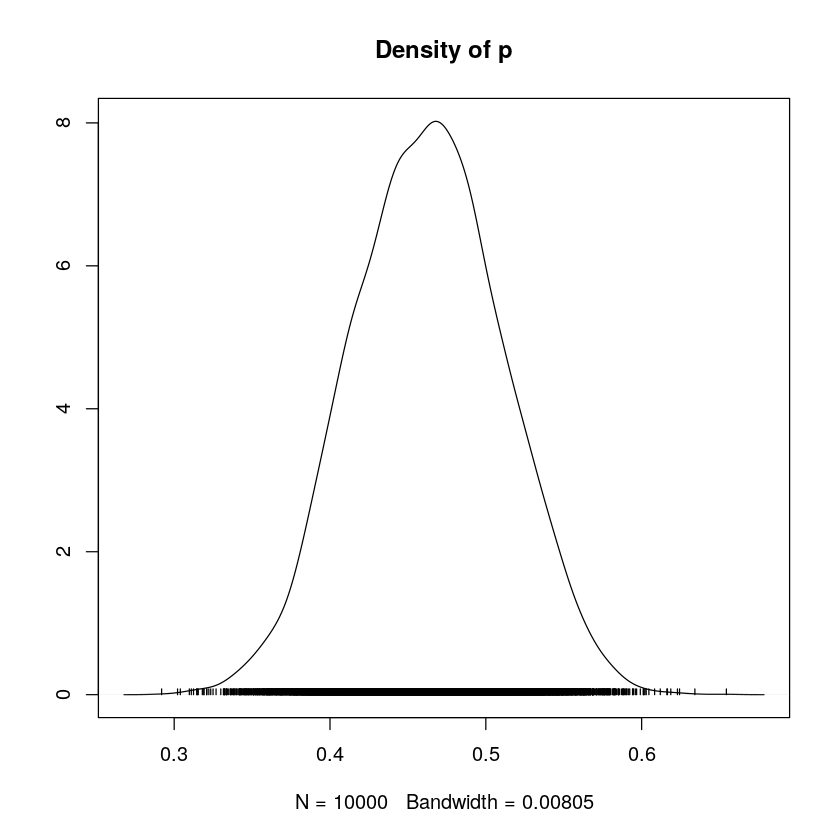Notice that after observing a poll in which $6$ of $10$ ($60\%$) of voters supported you, your updated posterior optimism and certainty about your underlying support, $p$, are slightly higher than they were prior to the poll.

# Updating the posterior¶

The posterior model of your underlying election support $p$ is informed by both the prior model of $p$ and polling data $X$. The code below reminds us of the posterior that evolved from the original prior ($Beta(45, 55)$) and original poll data ($X=6$ of $n=10$ polled voters support you).

In a 3-steps we will explore how using a different prior model or observing new data (or a combination of the two) might impact the posterior.

## ($X=6$, $n=10$)¶

Here we re-compile, simulate, and plot the $p$ posterior to reflect the setting in which we start with a $Beta(1,1)$ prior but observe the same polling data ($X=6$, $n=10$). NOTE: Recall that $Beta(1,1)$ is uniform across the $(0,1)$ interval.

In :
# COMPILE the model
vote_jags <- jags.model(textConnection(vote_model),
data = list(a = 1, b = 1, X = 6, n = 10),
inits = list(.RNG.name = "base::Wichmann-Hill", .RNG.seed = 100))

# SIMULATE the posterior
vote_sim <- coda.samples(model = vote_jags, variable.names = c("p"), n.iter = 10000)

# PLOT the posterior
plot(vote_sim, trace = FALSE, xlim = c(0,1), ylim = c(0,18))

Compiling model graph
Resolving undeclared variables
Allocating nodes
Graph information:
Observed stochastic nodes: 1
Unobserved stochastic nodes: 1
Total graph size: 5

Initializing model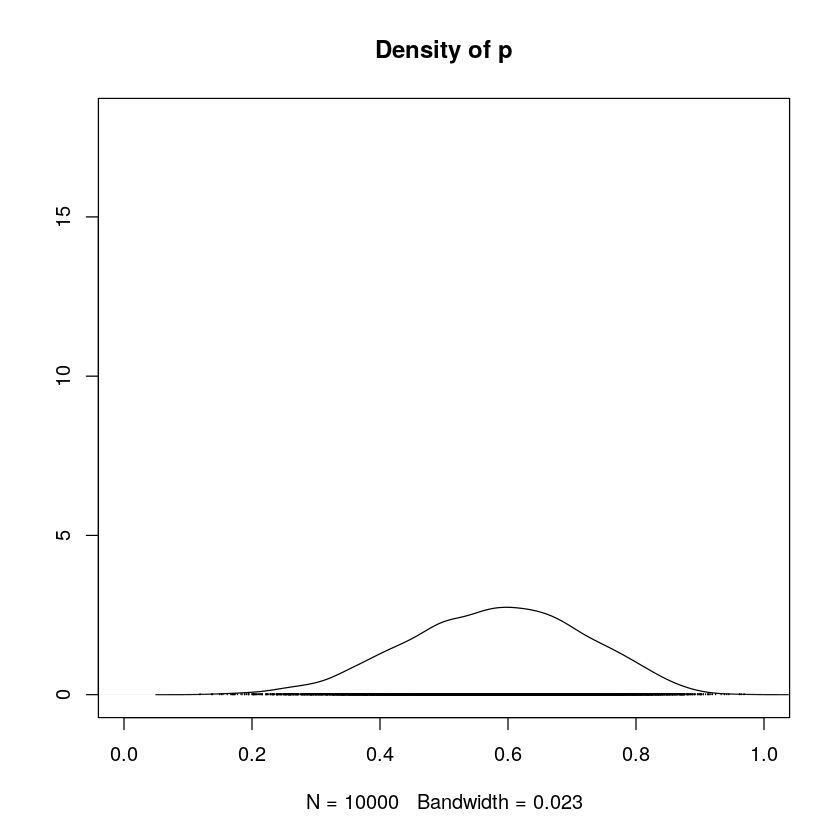## ($X=220$, $n=400$)¶

In a new poll, $214$ of $390$ voters support you. Combined with the first poll, a total $X=220$ of $n=400$ voters support you. We Re-compile, simulate, and plot the $p$ posterior to reflect these combined poll results and a $Beta(1,1)$ prior.

In :
# COMPILE the model
vote_jags <- jags.model(textConnection(vote_model),
data = list(a = 1, b = 1, X = 220, n = 400),
inits = list(.RNG.name = "base::Wichmann-Hill", .RNG.seed = 100))

# SIMULATE the posterior
vote_sim <- coda.samples(model = vote_jags, variable.names = c("p"), n.iter = 10000)

# PLOT the posterior
plot(vote_sim, trace = FALSE, xlim = c(0,1), ylim = c(0,18))

Compiling model graph
Resolving undeclared variables
Allocating nodes
Graph information:
Observed stochastic nodes: 1
Unobserved stochastic nodes: 1
Total graph size: 5

Initializing model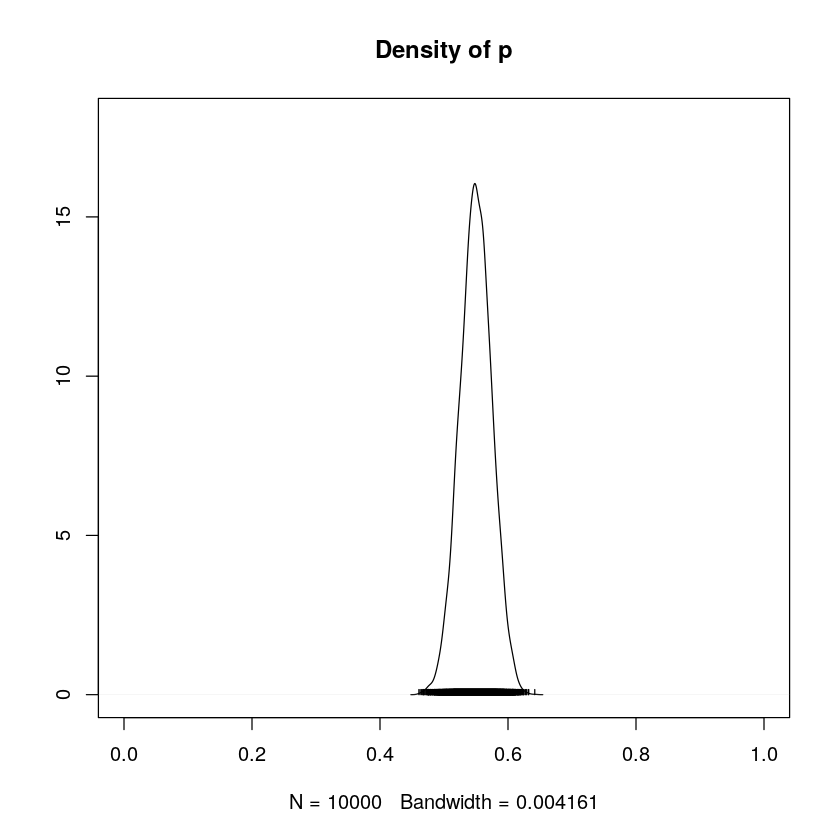## ($Beta(45,55)$) prior¶

Finally we, re-compile, simulate, and plot the $p$ posterior to reflect the combined poll results ($X=220$ of $n=400$) and our original $Beta(45,55)$ prior.

In :
# COMPILE the model
vote_jags <- jags.model(textConnection(vote_model),
data = list(a = 45, b = 55, X = 220, n = 400),
inits = list(.RNG.name = "base::Wichmann-Hill", .RNG.seed = 100))

# SIMULATE the posterior
vote_sim <- coda.samples(model = vote_jags, variable.names = c("p"), n.iter = 10000)

# PLOT the posterior
plot(vote_sim, trace = FALSE, xlim = c(0,1), ylim = c(0,18))

Compiling model graph
Resolving undeclared variables
Allocating nodes
Graph information:
Observed stochastic nodes: 1
Unobserved stochastic nodes: 1
Total graph size: 5

Initializing model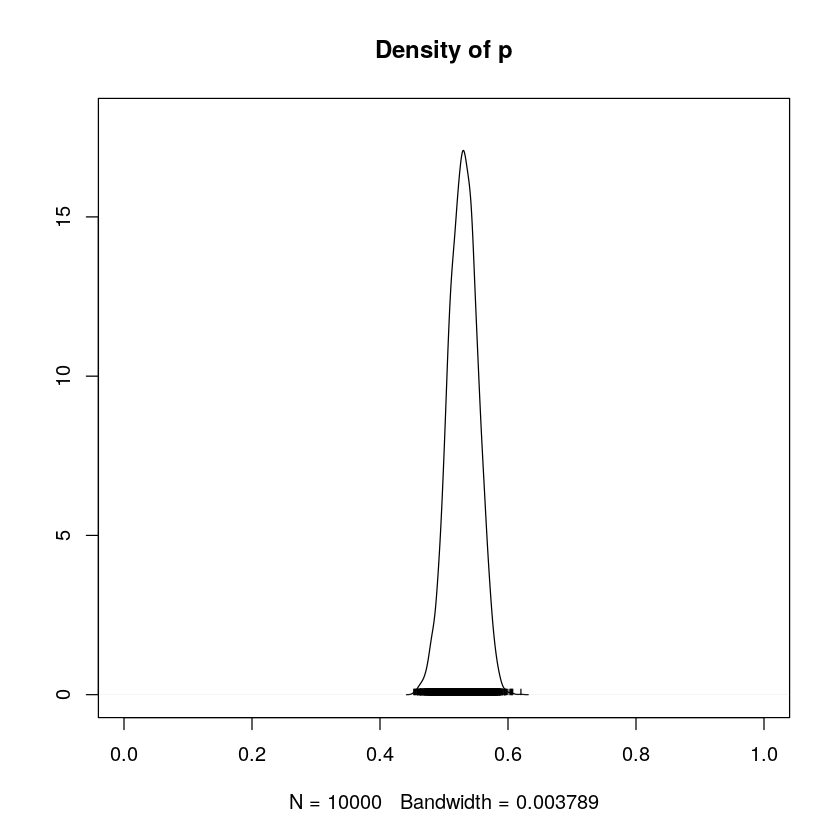• Even in light of the same data, different priors lead to different posteriors.

• The influence of the prior on the posterior diminishes as the sample size of your data increases.

• As sample size increases, your posterior understanding becomes more precise.

# The Normal-Normal Model¶

## Sleep deprivation¶

Research Question:

• How does sleep deprivation impact reaction time?

The Study

• measure reaction time on Day $0$
• restrict sleep to $3$ hours per night
• measure reaction time on Day $3$
• measure the change in reaction time

### Modeling change in reaction time¶

$Y_i$ = change in reaction time (ms)

Assume

• $Y_i$ are Normally distributed around some average change in reaction time $m$ with standard deviation $s$.
$$Y_i \sim N (m, s^2 )$$

#### Prior model for parameter $m$¶

• $Y_i$ = change in reaction time (ms)
• $Y_i \sim N (m, s^2 )$
• $m$ = average $Y_i$

Prior information:

• With normal sleep, average reaction time is $\sim 250$ ms
• Expect average to $\nearrow$ by $\sim 50$ ms
• Average is unlikely to $\searrow$ & unlikely to $\nearrow$ by more than $\sim 150$ ms

#### Prior model for parameter $s$¶

• $Y_i$ = change in reaction time (ms)
• $Y_i \sim N(m, s^2 )$
• $s$ = standard deviation of $Y_i$

Prior information:

• $s > 0$
• With normal sleep, s.d. in reaction times is $\sim 30$ ms
• $s$ is equally likely to be anywhere from $0$ to $200$ ms
##### The Normal-Normal Model¶

Likelihood:

• $Y_i \sim N(m, s^2 )$

Priors:

• $m \sim N(50, 25^2 )$
• $s \sim Unif(0, 200)$

# Normal-Normal priors¶

Researchers developed a test to evaluate the impact of sleep deprivation on reaction time. For subject $i$, let $Y_i$ be the change in reaction time (in ms) after $3$ sleep deprived nights. Of course, people react differently to sleep deprivation. It's reasonable to assume that $Y_i$ are Normally distributed around some average $m$ with standard deviation $s$: $Y_i \sim N(m,s^2)$.

In the first step of our Bayesian analysis, we'll simulate the following prior models for parameters $m$ and $s$: $m \sim N(50,252)$ and $s \sim Unif(0,200)$.

In :
# Take 10000 samples from the m prior
prior_m <- rnorm(n = 10000, mean = 50, sd = 25)

# Take 10000 samples from the s prior
prior_s <- runif(n = 10000, min = 0, max = 200)

# Store samples in a data frame
samples <- data.frame(prior_m, prior_s)

# Density plots of the prior_m & prior_s samples
#par(mfcol = c(2, 1))
ggplot(samples, aes(x = prior_m)) +
geom_density(color='darkblue', fill = 'lightblue') + theme_ridges()
ggplot(samples, aes(x = prior_s)) +
geom_density(color='darkblue', fill = 'lightblue') + theme_ridges()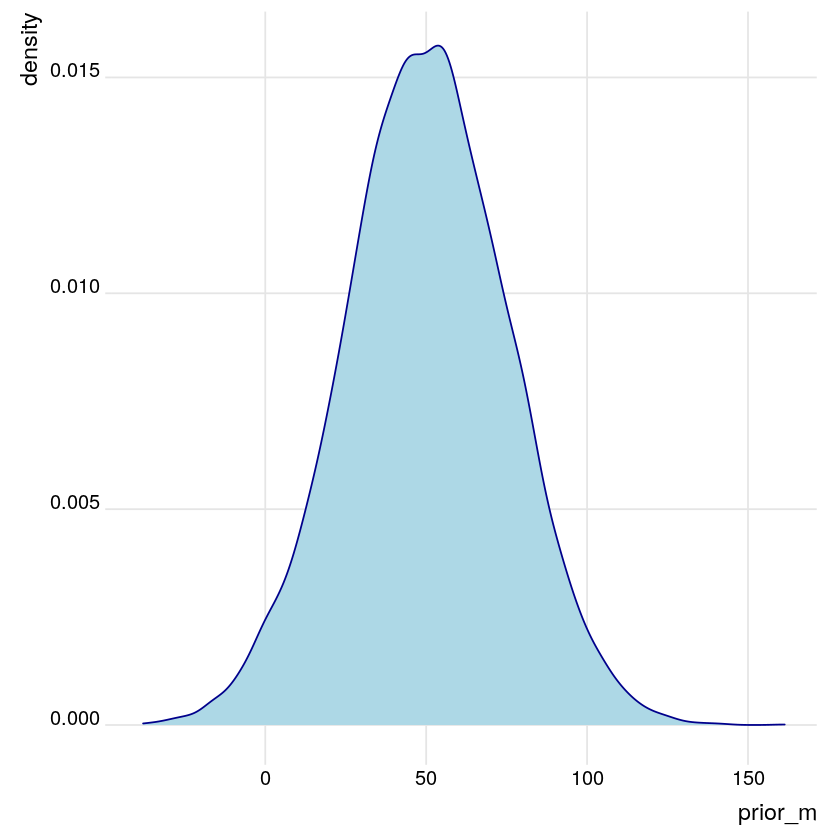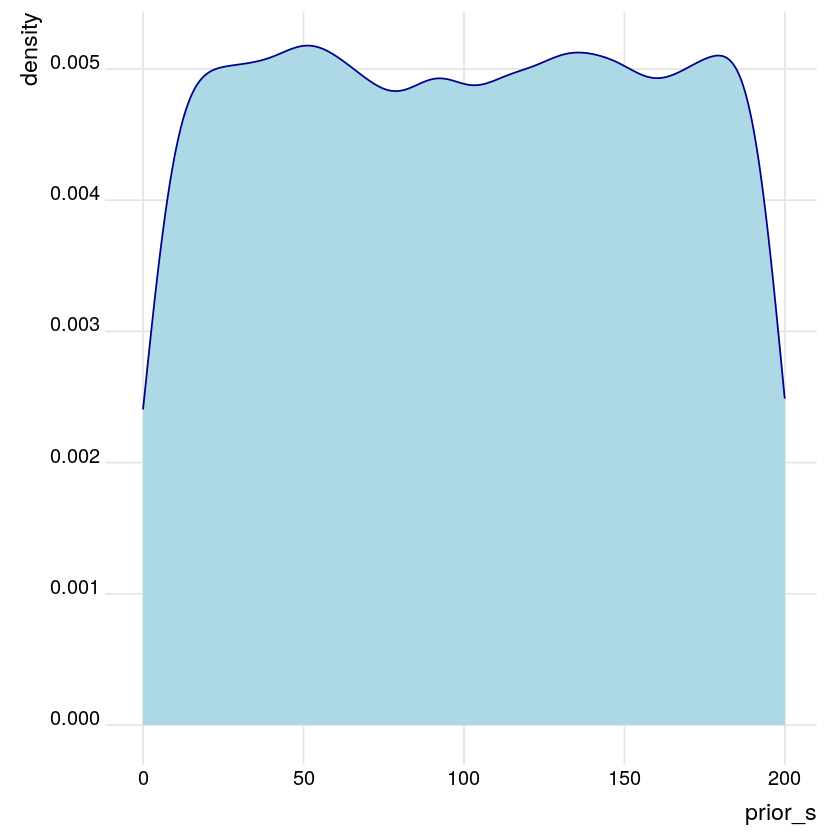# Sleep study data¶

Researchers enrolled $18$ subjects in a sleep deprivation study. Their observed sleep study data are loaded in the DataFrame sleep_study. These data contain the day_0 reaction times and day_3 reaction times after $3$ sleep deprived nights for each subject.

We will define and explore diff_3, the observed difference in reaction times for each subject.

In :
# Check out the first 6 rows of sleep_study

# Define diff_3
sleep_study <- sleep_study %>%
mutate(diff_3 = day_3 - day_0)

# Histogram of diff_3
ggplot(sleep_study, aes(x = diff_3)) +
geom_histogram(binwidth = 20, color='darkblue', fill = 'lightblue') + theme_ridges()

# Mean and standard deviation of diff_3
sleep_study %>%
summarize(mean(diff_3), sd(diff_3))

A data.frame: 6 × 3
subjectday_0day_3
<int><dbl><dbl>
1308249.5600321.4398
2309222.7339204.7070
3310199.0539232.8416
4330321.5426285.1330
5331287.6079320.1153
6332234.8606309.7688
A data.frame: 1 × 2
mean(diff_3)sd(diff_3)
<dbl><dbl>
26.3402137.20764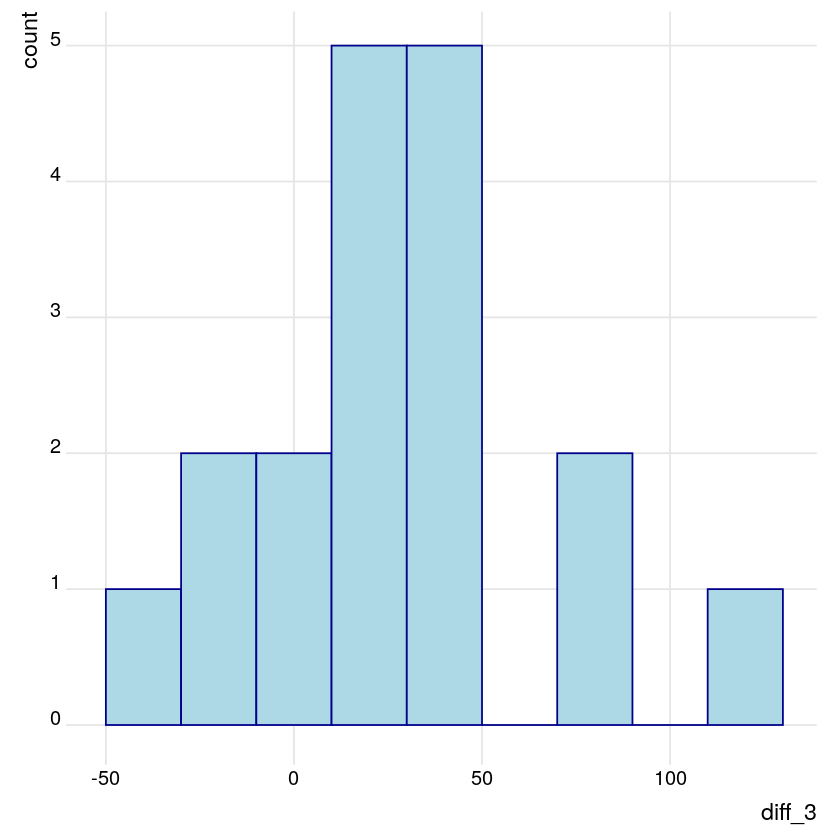Reaction times increased by an average of $\sim 26$ ms with a standard deviation of $\sim 37$ ms. Further, only $4$ of the $18$ test subjects had faster reaction times on day $3$ than on day $0$.

## DEFINE the Normal-Normal for RJAGS¶

• $Y_i \sim N(m, s^2 )$ for $i$ in $1, 2, \dots , 18$
• NOTE: precision = $variance^{−1}$ = $s^{−2}$
• $m \sim N(50, 25^2)$
• $s \sim Unif(0, 200)$

## Define, compile, & simulate the Normal-Normal¶

Upon observing the change in reaction time $Y_i$ for each of the $18$ subjects $i$ enrolled in the sleep study, WE can update our posterior model of the effect of sleep deprivation on reaction time. This requires the combination of insight from the likelihood and prior models:

• likelihood: $Y_i \sim N(m,s^2)$
• Priors: $m \sim N(50,252)$ and $s \sim Unif(0,200)$
In :
# DEFINE the model
sleep_model <- "model{
# Likelihood model for Y[i]
for(i in 1:length(Y)) {
Y[i] ~ dnorm(m, s^(-2))
}

# Prior models for m and s
m ~ dnorm(50, 25^(-2))
s ~ dunif(0, 200)
}"

# COMPILE the model
sleep_jags <- jags.model(
textConnection(sleep_model),
data = list(Y = sleep_study$diff_3), inits = list(.RNG.name = "base::Wichmann-Hill", .RNG.seed = 1989) ) # SIMULATE the posterior sleep_sim <- coda.samples(model = sleep_jags, variable.names = c("m", "s"), n.iter = 10000) # PLOT the posterior plot(sleep_sim, trace = FALSE)  Compiling model graph Resolving undeclared variables Allocating nodes Graph information: Observed stochastic nodes: 18 Unobserved stochastic nodes: 2 Total graph size: 28 Initializing model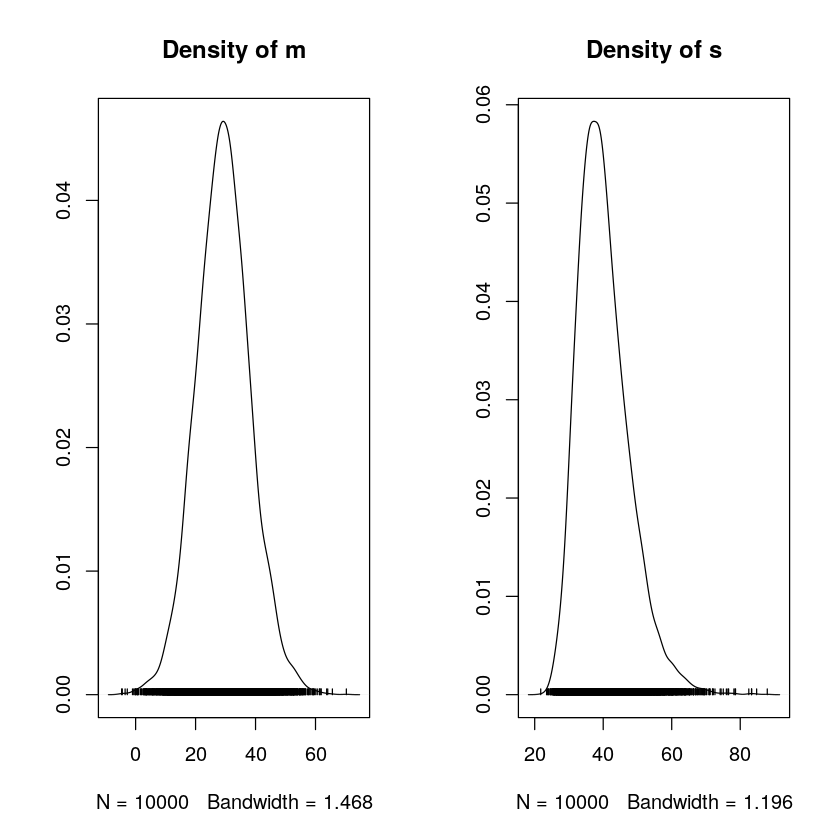We're more certain that average reaction time increases but that the magnitude of the increase is less than assumed prior to observing the data. # Markov chains¶ Markov chains are stochastic models that describe sequences of possible events. Each event comes from a set of outcomes, and each outcome determines which outcome occurs next, according to a fixed set of probabilities. An important feature of Markov chains is that they are memoryless: everything that you would possibly need to predict the next event is available in the current state, and no new information comes from knowing historical events. • RJAGS goal: Utilize Markov chains to approximate posteriors that are otherwise too complicated to define or sample • The$m$Markov chain traverses the sample space of$m$, mimics a random sample, and converges to the posterior. ## Storing Markov chains¶ Let$m$be the average change in reaction time after$3$days of sleep deprivation. Previously we obtained an approximated a sample of$10,000$draws from the posterior model of$m$. We stored the resulting mcmc.list object as sleep_sim. The sample of$m$values in sleep_sim is a dependent Markov chain, the distribution of which converges to the posterior. In : # Check out the head of sleep_sim head(sleep_sim) # Store the chains in a data frame sleep_chains <- data.frame(sleep_sim[], iter = 1:10000) # Check out the head of sleep_chains head(sleep_chains)  [] Markov Chain Monte Carlo (MCMC) output: Start = 1001 End = 1007 Thinning interval = 1 m s [1,] 17.25796 31.46256 [2,] 34.58469 37.88655 [3,] 36.45480 39.58056 [4,] 25.00971 39.69494 [5,] 29.95475 35.90001 [6,] 28.43894 37.46466 [7,] 38.32427 35.44081 attr(,"class")  "mcmc.list" A data.frame: 6 × 3 msiter <dbl><dbl><int> 117.2579631.462561 234.5846937.886552 336.4548039.580563 425.0097139.694944 529.9547535.900015 628.4389437.464666 ## Markov chain trace plots¶ A trace plot provides a visualization of a Markov chain's longitudinal behavior. Specifically, a trace plot for the$m$chain plots the observed chain value (y-axis) against the corresponding iteration number (x-axis).We will construct trace plots of the$m$chain. In : # Use plot() to construct trace plots of the m and s chains plot(sleep_sim, density = FALSE) # Use ggplot() to construct a trace plot of the m chain ggplot(sleep_chains, aes(x = iter, y = m)) + geom_line() + theme_ridges() # Trace plot the first 100 iterations of the m chain ggplot(sleep_chains[1:100, ], aes(x = iter, y = m)) + geom_line() + theme_ridges()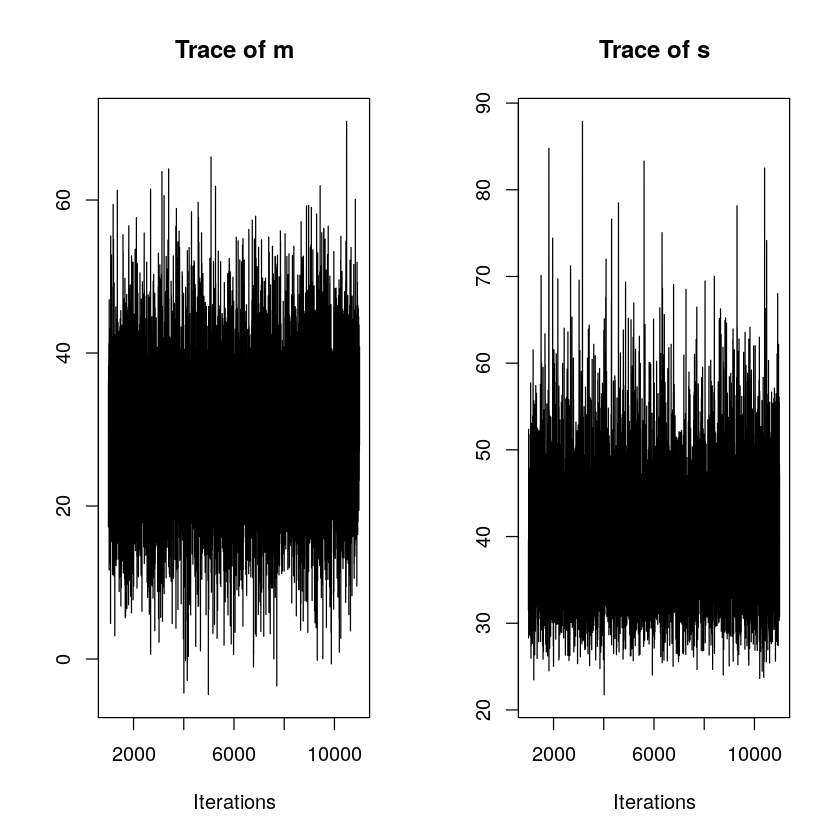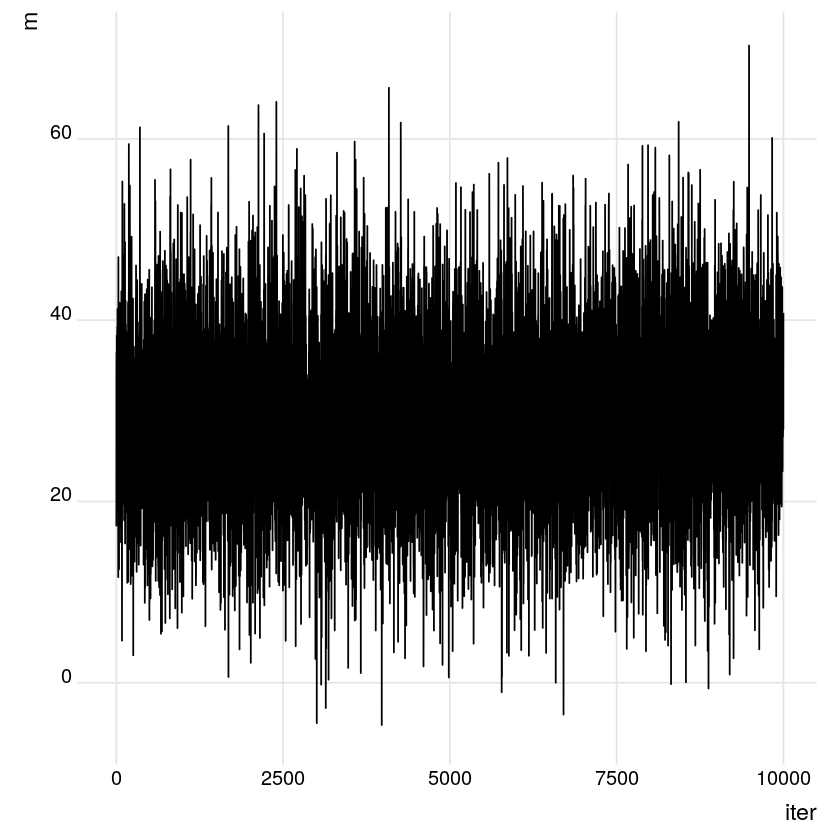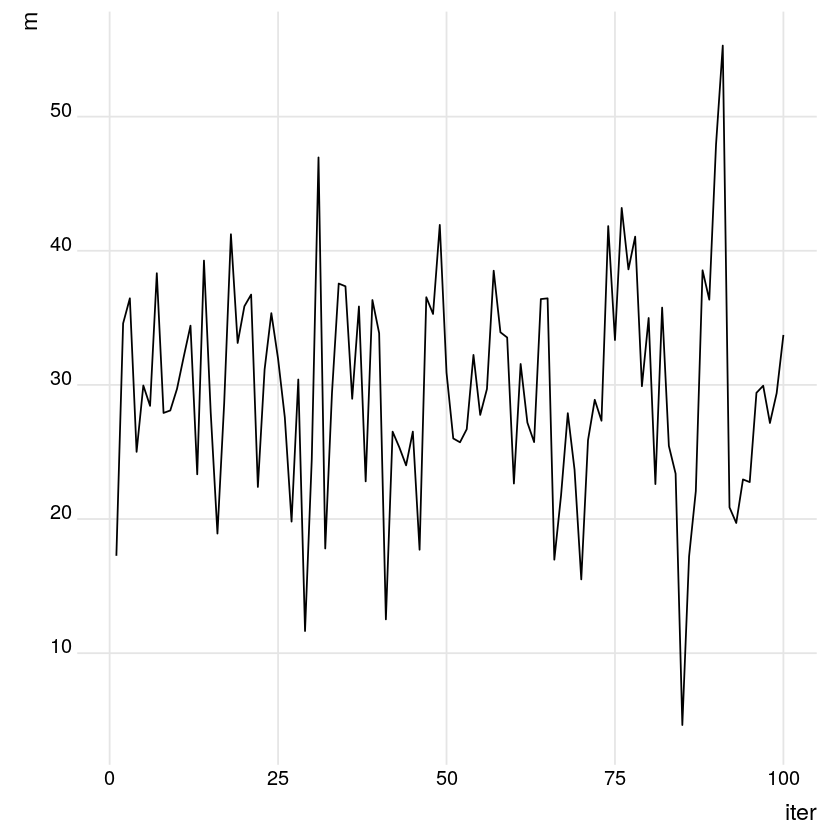Note that the longitudinal behavior of the chain appears quite random and that the trend remains relatively constant. This is a good thing - it indicates that the Markov chain (likely) converges quickly to the posterior distribution of$m$. ## Markov chain density plots¶ Whereas a trace plot captures a Markov chain's longitudinal behavior, a density plot illustrates the final distribution of the chain values. In turn, the density plot provides an approximation of the posterior model. We will construct and examine density plots of the$m\$ Markov chain.

In :
# Use plot() to construct density plots of the m and s chains
plot(sleep_sim, trace = FALSE)

# Use ggplot() to construct a density plot of the m chain
ggplot(sleep_chains, aes(x = m)) +
geom_density(color='darkblue', fill = 'lightblue') + theme_ridges()